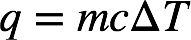# Problem: Evaporating sweat cools the body because evaporation is an endothermic process: H2O(l) → H2O(g); ΔH˚rxn= + 44.01 kJ. Estimate the mass of water that must evaporate from the skin to cool the body by 0.55˚C. Assume a body mass of 95 kg and assume that the specific heat capacity of the body is 4.0 J/g•˚C.

###### FREE Expert Solution

Recall: The amount of heat needed to be absorbed or released to change the temperature of an object is given by:where m = mass of the object(in g), c = specific heat capacity (in J/g • ˚C), and ΔT = change in temperature (in ˚C).

98% (443 ratings)###### Problem Details

Evaporating sweat cools the body because evaporation is an endothermic process: H2O(l) → H2O(g); ΔH˚rxn= + 44.01 kJ. Estimate the mass of water that must evaporate from the skin to cool the body by 0.55˚C. Assume a body mass of 95 kg and assume that the specific heat capacity of the body is 4.0 J/g•˚C.